# face recognition[Euclidean-distance-based loss][FaceNet]

### 0 引言

• 相同ID的人脸距离较小；
• 不同ID的人脸距离较大。

• 做预测的时候，期望bottleneck表征能够很好的对新人脸进行泛化；
• 通过使用该bottleneck层，每个人脸得到的特征向量维度是很大的（比如1k维）。虽然有工作基于PCA做特征约间，但是PCA是一个线性模型。

FaceNet使用训练后的深度卷积网络直接对嵌入向量进行优化，而不是如之前的DL方法一样去优化所谓的bottleneck层。FaceNet基于《Distance metric learning for large margin nearest neighbor classification》采用triplet loss进行训练，并输出只有128维的embedding向量。这里的triplet包含人脸三元组（即三张人脸图片$a,b,c$，其中$a,b$是来自同一个ID的人脸，$c$是其他ID的人脸），loss的目的就是通过一个距离边际去划分正对和负样本。这里人脸图片是只包含人脸区域的图片块，并不需要严格的2D或者3D的对齐，只需要做尺度缩放和平移即可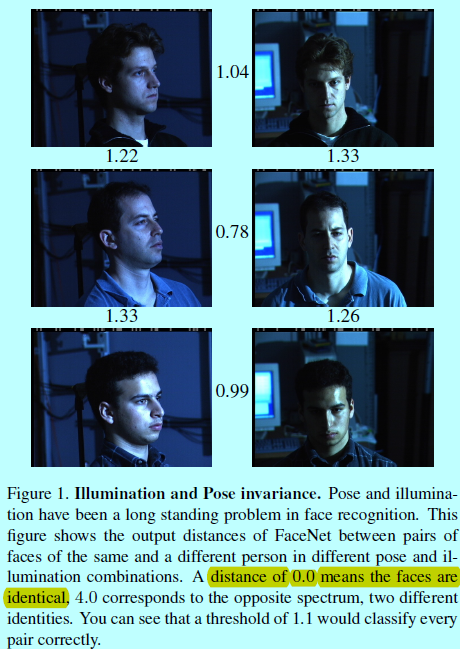### 1 FaceNet

• 基于《Visualizing and understanding convolutional networks》的深度网络（包含多个交错的卷积层，非线性激活，局部响应归一化（local response normalizations）和最大池化层），增加了几个额外的1x1xd卷积层；
• 基于Inception模型。

FaceNet采用了一个深度卷积网络，这部分有2种选择（上述两种），整体结构如下图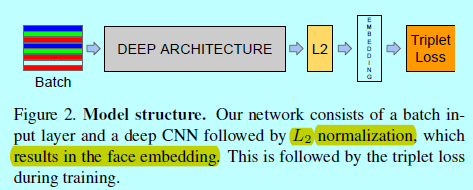#### 1.1 triplet loss

embedding表示为$f(x)\in R^d$。通过将一个图片$x$嵌入到d维欧式空间中。另外，限制这个embedding，使其处在d维超球面上，即$||f(x)||_2=1$。这里要确保一个特定人的图片$x_i^a$(锚)和该人的其他图片$x_i^p$(正样本)相接近且距离大于锚与其他人的图片$x_i^n$(负样本)。如下图所示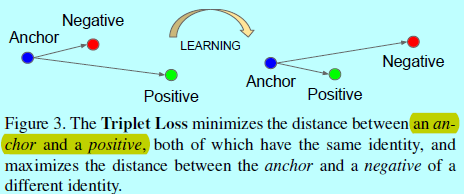$||f(x_i^a)-f(x_i^p)||_2^2+\alpha < ||f(x_i^a)-f(x_i^n)||_2^2,\, \, \forall (f(x_i^a),f(x_i^p),f(x_i^n))\in T$

$\sum_i^N\left [ ||f(x_i^a)-f(x_i^p)||_2^2-||f(x_i^a)-f(x_i^n)||_2^2+\alpha\right ]_+$

#### 1.2 triplet 选择

• 每隔$n$步离线的生成triplets，使用最近的网络checkpoint，用其基于训练集的子集计算$\arg min$$\arg max$
• 在线生成triplet，这通过一个mini-batch内部选择硬正/负样本对来实现。

$||f(x_i^a)-f(x_i^p)||_2^2<||f(x_i^a)-f(x_i^n)||_2^2$

#### 1.3 深度卷积网络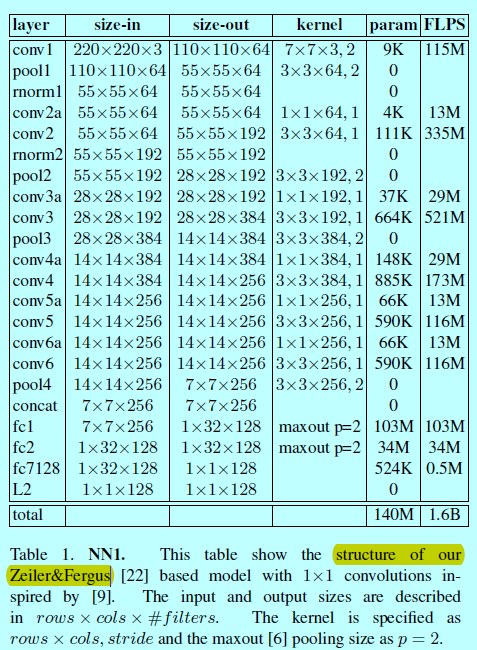• NNS1只有26M参数量，需要220M FLOPS的计算；
• NNS2有4.3M参数量，且只需要20M FLOPS计算量。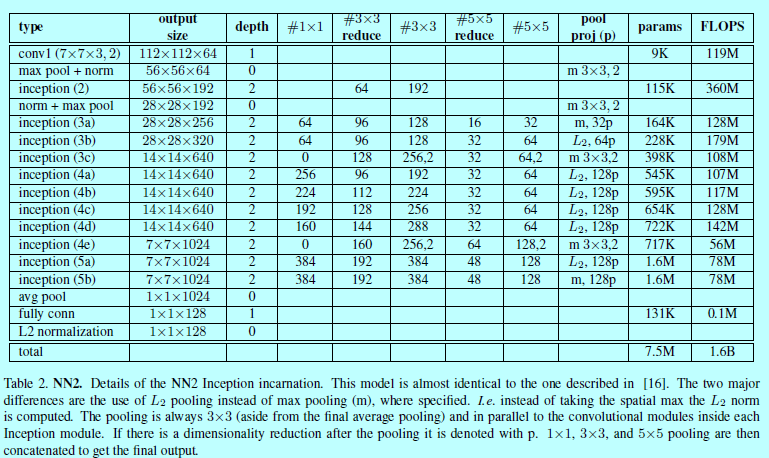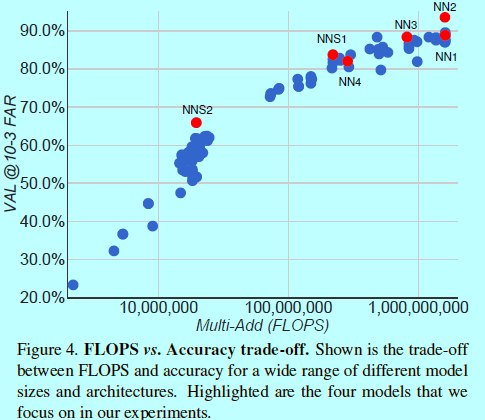### 2 实验及分析

......

posted @ 2018-12-10 20:33  仙守  阅读(1092)  评论(0编辑  收藏  举报